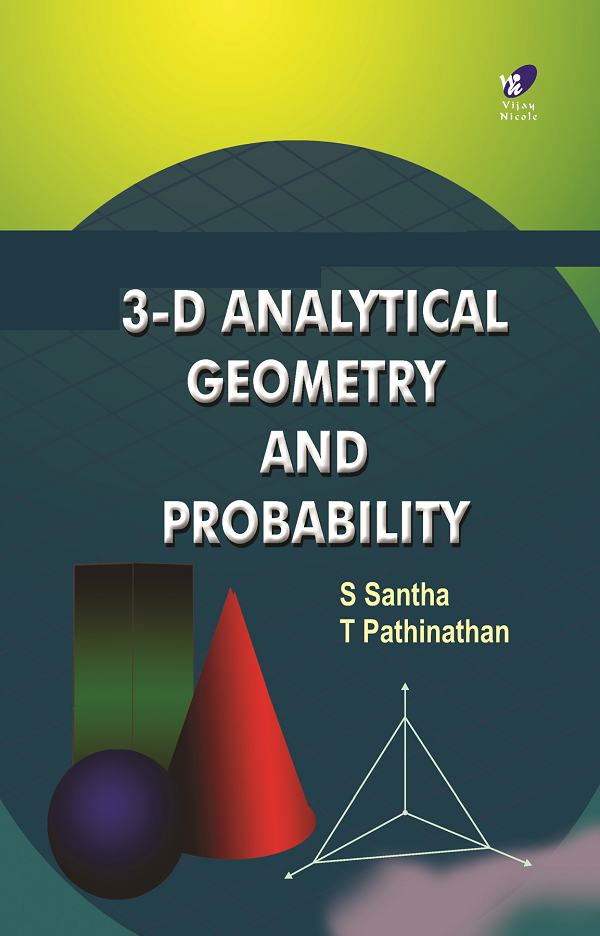3-D Analytical Geometry and Probability
Author(s):
Subject(s):
ISBN 9789394681583
Published Date
Pages 294
Description

This book offers a graduate level exposition of selected topics in 3-D Analytical Geometry and Probability. It is designed to cover the requirements of the new syllabi for second year B.Sc. Mathematics students of all universities in Tamil Nadu. Even a beginner who likes to get an expertise in Solid Geometry and Probability Theory can find this book very useful and enriching.

Cover
Title Page
Preface
Contents
Part I 3-D Analytical Geometry
Chapter 1 Introduction
Introduction
Coordinate Axes and Coordinate Planes
Coordinates of a Point
Distance Between Two Points
Definition—Tetrahedron
Angle Between Two Lines
Direction Angles and Direction Cosines of a Line
Definition—Direction Cosines
Direction Cosines of Coordinate Axes
Relation Between Direction Cosines of a Straight Line
Direction Ratios
Direction Ratios of the Line Joining Two Points
Angle Between Two Lines
Condition for Parallelism and Perpendicularity of Two Lines
Chapter 2 The Plane
Plane
Definition
General Form of the Equation of a Plane
Intercept Form
Normal Form
Angle Between Two Planes
Equation of a Plane Passing through the Line of Intersection of Two Given Planes
Chapter 3 The Straight Lines
General Form of Equation of a Line
Symmetrical Form of the Equations of a Line
Transformation of General Form into Symmetrical Form
The Plane and the Straight Line
Angle Between a Line and a Plane
A Line of Greatest Slope in a Plane
Coplanar Lines
First Method
Second Method
Lines Intersecting Two Given Lines
Skew Lines
Definition—Skew Lines
Shortest Distance Between the Lines
Definition
Chapter 4 The Sphere
Definition
Equation of a Sphere whose Centre and Radius are Given
General Form of Equation of a Sphere
Plane Section of a Sphere
Equation of a Circle
Equation of a Sphere Passing through a Given Circle
The Intersection of Two Spheres
Tangent and Tangent Plane
Equation of the Tangent Plane to a Sphere
Length of the Tangent from an External Point to a Sphere
Orthogonal Spheres
Condition for the Two Spheres to Cut Orthogonally
Part II Probability
Chapter 5 Introduction
Historically
Basic Terms
Measurement of Probability
Classical Approach
Axiomatic Approach to Probability
Probability Function
Set Theoretic Notations
Boole’s Inequality
Conditional Probability
Some Results on Independent Events
Multiplication Theorem on Probability
Multiplication Theorem of Probability for Independent Events
Some Theorems on Conditional Probability
Baye’s Theorem
Chapter 6 Random Variables
Introduction
Random Variable (R.V.)
Distribution Function
Discrete Random Variables
Continuous Random Variables
Two-dimensional Random Variables
Independent Random Variables
Chapter 7 Mathematical Expectation
Definition
Properties of Expectation
Multiplication Theorem of Expectation
Moment, Mean and Standard Deviation
Chapter 8 Moment and Probability Generating Functions
Moment Generating Functions
Definition
Deficiencies of Moment Generating Functions
Properties of Moment Generating Function
Standard Variate
Chebychev’s Inequality
Probability Generating Function
Definition
Probability Generating Function for Two Variables X1 and X2
Simple Results on Probability Generating Function
Probability Generating Function for Different Distributions

Dr S Santha is currently Lecturer, Department of Mathematics, Sri Meenakshi Government College for Women, Madurai.Prior to this,she was the Head,Department of Mathematics , Noorul Islam College of Engineering , Kanyakumari . She has over 21 years of experience in teaching Mathematics.

T Pathinathan is Senior Scale Lecturer, Department of Mathematics, Loyola College, Chennai. He has 16 years of experience in teaching mathematics at various levels.